#### Distributions

##### Introduction
Commonly encountered distributions are built into BUGS, as described on this page. Further distributions are included in GeoBUGS and ReliaBUGS: see the Spatial distributions, Temporal distributions and Reliability distributions pages for details of these.

If a distribution is not built into BUGS, distributions specified by a log-likelihood can also be used: see Generic sampling distributions for details.

##### Binomial

The Binomial distribution is defined by the pmf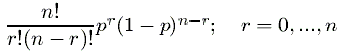In the BUGS language it is used as

`r ~ dbin(p, n)`

##### Binomial

The Binomial distribution is defined by the pmfIn the BUGS language it is used as

`r ~ dbin(p, n)`

##### Categorical

The Categorical distribution is defined by the pmf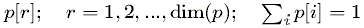In the BUGS language it is used as

`r ~ dcat(p[])`

##### Negative Binomial
The Negative Binomial distribution is defined by the pmf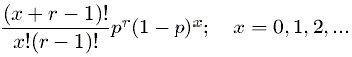In the BUGS language it is used as

`x ~ dnegbin(p, r)`

##### Poisson
The Poisson distribution is defined by the pmf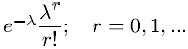In the BUGS language it is used as

`r ~ dpois(lambda)`

##### Non-central hypergeometric
The Non-central hypergeometic distribution is defined by the pmf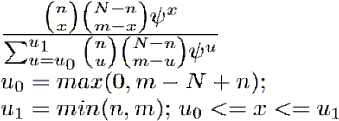In the BUGS language it is used as

`x ~ dhyper(n, m, N, psi)`

##### Beta
The Beta distribution is defined by the pdf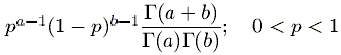In the BUGS language it is used as

`p ~ dbeta(a, b)`

##### Chi-squared
The Chi-squared distribution is defined by the pdf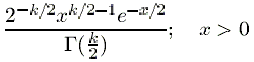In the BUGS language it is used as

`x ~ dchisqr(k)`

##### Double Exponential
The Double Exponential distribution is defined by the pdfIn the BUGS language it is used as

`x ~ ddexp(mu, tau)`

##### Exponential
The Exponential distribution is defined by the pdf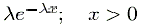In the BUGS language it is used as

`x ~ dexp(lambda)`

##### Flat
The improper Flat distribution has a constant value for all x. It is not a proper distribution.

In the BUGS language it is used as

`x ~ dflat()`

##### Gamma
The Gamma distribution is defined by the pdf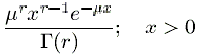In the BUGS language it is used as

`x ~ dgamma(r, mu)`

##### Generalized extreme value
The Generlized extreme value distribution is defined by the pdf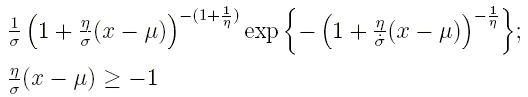In the BUGS language it is used as

`x ~ dgev(mu, sigma, eta)`

##### Generalized F
The Generalized F distribution is defined by the pdf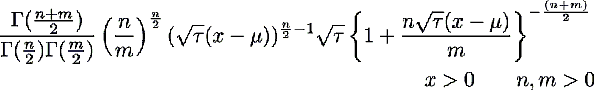It reduces to the standard F for mu=0, tau=1. In the BUGS language it is used as

`x ~ df(n, m, mu, tau)`

##### Generalized Gamma
The Generalized Gamma distribution is defined by the pdf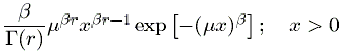In the BUGS language it is used as

`x ~ dggamma(r, mu, beta)`

##### Generalized Pareto
The Generalized Pareto distribution is defined by the pdf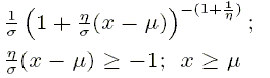In the BUGS language it is used as

`x ~ dgpar(mu, sigma, eta)`

##### Generic log-likelihood distribution
The generic log-likelihood distribution is defined by the pdf exp(lambda). It allows generic log-likelihoods to be used in BUGS. See Generic sampling distributions for details. Note it does not depend on x.

In the BUGS language it is used as

`x ~ dloglik(lambda)`

##### Log-normal
The Log-normal distribution is defined by the pdf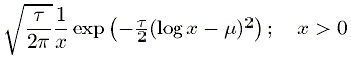In the BUGS language it is used as

`x ~ dlnorm(mu, tau)`

##### Logistic
The Logistic distribution is defined by the pdf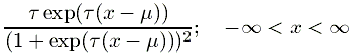In the BUGS language it is used as

`x ~ dlogis(mu, tau)`

##### Normal
The Normal distribution is defined by the pdf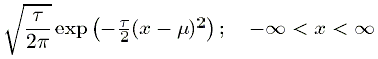In the BUGS language it is used as

`x ~ dnorm(mu, tau)`

##### Pareto
The Pareto distribution is defined by the pdf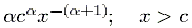In the BUGS language it is used as

`x ~ dpar(alpha, c)`

##### Student-t
The Student-t distribution is defined by the pdf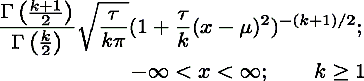In the BUGS language it is used as

`x ~ dt(mu, tau, k)`

##### Uniform
The Uniform distribution is defined by the pdf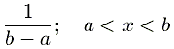In the BUGS language it is used as

`x ~ dunif(a, b)`

##### Weibull
The Weibull distribution is defined by the pdf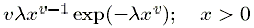In the BUGS language it is used as

`x ~ dweib(v, lambda)`

##### Multinomial
The Multinomial distribution is defined by the pmf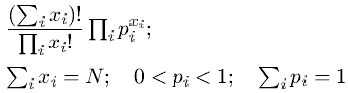In the BUGS language it is used as

`x[] ~ dmulti(p[], N)`

##### Dirichlet
The Dirichlet distribution is defined by the pdf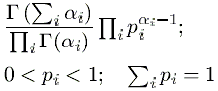In the BUGS language it is used as

`p[] ~ ddirich(alpha[])`

It may also be spelt `ddirch` as in WinBUGS.

##### Multivariate normal
The Multivariate Normal distribution is defined by the pdf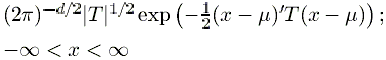In the BUGS language it is used as

`x[] ~ dmnorm(mu[], T[,])`

##### Multivariate Student-t
The Multivariate Student-t distribution is defined by the pdf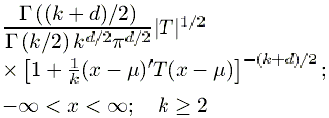In the BUGS language it is used as

`x[] ~ dmt(mu[], T[,], k)`

##### Wishart
The Wishart distribution is defined by the pdf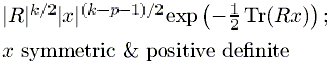In the BUGS language it is used as

`x[,] ~ dwish(R[,], k)`